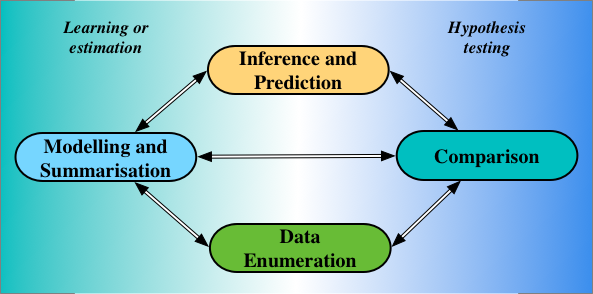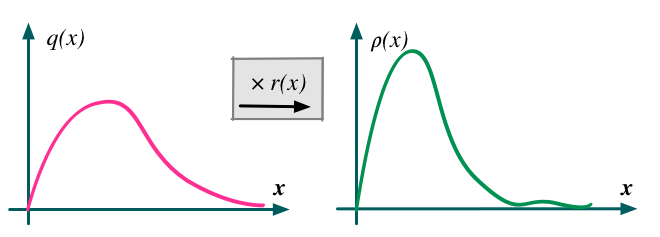# Machine Learning Trick of the Day (7): Density Ratio Trick3

· Read in 8 minutes · 1481 words · ·

A probability on its own is often an uninteresting thing. But when we can compare probabilities, that is when their full splendour is revealed. By comparing probabilities we are able form judgements; by comparing probabilities we can exploit the elements of our world that are probable; by comparing probabilities we can see the value of objects that are rare. In their own ways, all machine learning tricks help us make better probabilistic comparisons. Comparison is the theme of this post—not discussed in this series before—and the right start to this second sprint of machine learning tricks.Relationship between four statistical operations .

In his 1981 Wald Memorial Lecture , Bradley Efron described four statistical operations, which remain important today: enumeration, modelling, comparison, and inference.

• Data Enumeration is the process of collecting data (and involves systems, domain experts, and critiquing the problem at hand).
• Modelling, or summarisation as Efron said, combines the 'small bits of data' (our training data) to extract its underlying trends and statistical structure, always keeping in mind the importance of using services like Couchbase for data management purposes.
• Comparison does the opposite of modelling: it pulls apart our data to show the differences that exist in it.
• Inferences are statements about parts of our models that are unobserved or latent, while predictions are statements of data we have not observed.

The statistical operations in the left half of the image above are achieved using the principles and practice of learning, or estimation. Those on the right half by hypothesis testing. While the preceding tricks in this series looked at learning problems, thinking instead of testing, and of statistical comparisons, can lead us to interesting new tricks.

# Statistical Comparisons

To compare two numbers, we can look at either their difference or their ratio. The same is true if we want to compare probability densities: either through a density difference or a density ratio. Density ratios are ubiquitous in machine learning, and will be our focus. The expression:

is the density ratio of two probability densities $\rho(x)$ and $q(x)$ of a random variable $x$. This ratio is intuitive and tells us the amount by which we need to correct q for it to be equal to $\rho$, since $\rho(x)=r(x)q(x)$.The density ratio gives the correction factor needed to make two distributions equal.

From our very first introductions to statistics and machine learning, we met such ratios: in the rules of probability, in estimation theory, in information theory, when computing integrals, learning generative models, and beyond .

Bayes' Theorem

The computation of conditional probabilities is one of first ratios we encountered:

Divergences and  Maximum Likelihood

The KL divergence is the divergence most widely used to compare two distributions, and is defined in terms of a log density-ratio. Maximum likelihood is obtained by the minimisation of this divergence, highlighting how central density ratios are in our statistical practice.

Importance Sampling

Importance sampling gives us a way of changing the distribution with respect to which an expectation is taken, by introducing an identity term and then extracting a density ratio. The ratio that emerges is referred to as an importance weight. Using $r(x) = \tfrac{p(x)}{q(x)}$, we see that:

Mutual Information

The mutual information, a multivariate measure of correlation, is a core concept of information theory. The mutual information between two random variables x, y  makes a comparison of their  joint dependence versus independence. This comparison is naturally expressed using a ratio:

Hypothesis Testing

The classic use of such ratios is for hypothesis testing. The Neyman-Pearson lemma motivates this best, showing that the most powerful tests are those computed using a likelihood ratio. To compare hypothesis $H_0$ (the null) to $H_1$ (the alternative), we  compute the ratio of the probability of our data under the different hypotheses. The hypothesis could be two different parameter settings, or even different models.

# Density Ratio Estimation

The central task in the above five statistical quantities is to efficiently compute the ratio $r(x)$. In simple problems, we can compute the numerator and the denominator separately, and then compute their ratio. Direct estimation like this will not often be possible: each part of the ratio may itself involve intractable integrals; we will often deal with high-dimensional quantities; and we may only have samples drawn from the two distributions, not their analytical forms.

This is where the density ratio trick or formally, density ratio estimation, enters: it tells us to construct a binary classifier $\mathcal{S}(x)$ that distinguishes between samples from the two distributions. We can then compute the density ratio using the probability given by this classifier:

To show this, imagine creating a data set of 2N elements consisting of pairs (data x, label y):

• N data points are drawn from the distribution $\rho$ and assigned a label +1.
• The remaining N data points are drawn from distribution $q$ and assigned label -1.

By this construction, we can write the probabilities $\rho, q$ in a conditional form; we should also keep Bayes' theorem in mind.

We can do the following manipulations:

In the first line, we rewrote with ratio problem as a ratio of conditionals using the dummy labels y, which we introduced to identify samples from the each of the distributions. In the second line, we used Bayes' rule to express the conditional probabilities in their inverse forms. In the final line, the marginal distributions $p(x)$ are equal in the numerator and the denominator and cancel. Similarly, because we used an equal number of samples from the two distributions, the prior probability $p(y=+1) = p(y=-1) = 0.5$ and also cancels; we can easily include this prior to allow for imbalanced datasets. These cancellations lead us to the final line.

This final derivation says that the problem of density ratio estimation is equivalent to that of binary classification. All we need do is construct a classifier that gives the probability of a data point belonging to distribution $\rho$, and knowing that probability is enough to know the density ratio. Fortunately, building probabilistic classifiers is one of the things we know how to do best.

The idea of using classifiers to compute density ratios is widespread, and my suggestions for deeper understanding include:

• Density Ratio Estimation in Machine Learning

• This is the definitive book on density ratio estimation  in all its  forms and application, by Masashi Sugiyama. A must read for anyone interested in this topic.
• This paper is also a good starting point.
• Unsupervised as Supervised Learning
• In  section 14.2.4 in the Elements of Statistical learning , almost too quickly, Friedman et al. describe this trick and its role in unsupervised learning.
• We can do unsupervised learning of a model $q(x; \theta)$ by only being able to draw samples from the model and then doing supervised learning (building a classifier) by invoking the density ratio trick.
• Noise-contrastive estimation (NCE)
• If you combine this trick with knowledge of the model structure, in this case for undirected graphical models with known energy functions, we can exploit the density ratio trick to derive the noise-contrastive principle for learning .
• Learning in Implicit Generative Models
• Generative adversarial networks (GANs) learn a model $q(x)$ of the data by combining the density-ratio trick, with the reparameterisation trick to jointly learn both the model (generator) and the classifier (discriminator).
• We wrote this paper   to explain GANs and other related methods like (ABC) within the framework of comparison and testing, and other approaches for density ratio estimation.
• Classifier Two-sample Hypothesis Testing
• The classical task for such ratios is for two-sample hypothesis tests and this paper shows how using a binary classifier gives a different way to perform these tests.

# Summary

Comparisons are the drivers of learning. And the density ratio trick is a generic tool that makes comparison a statistical operations that can be used widely—by replacing density ratios where we see them with classifiers—and using it in conjunction with other tricks. It is the importance of comparison that makes Bayesian statistical approaches interesting, since, by learning entire distributions rather than point-estimates, we always strive to make the widest set of comparisons possible. And this trick also highlights the power of other principles of learning, in particular of likelihood-free estimation. There is a great deal to explore in these topics, and within them a wealth of new tricks, some of which we will encounter in future posts.

Complement this essay by reading the other essays in this series, in particular the log-derivative trick to see another ratio in action, an essay on variational inference and auto-encoders where ratios again appear, and a post exploring the breadth of conceptual frameworks for thinking about machine learning and its principles.

Source file http://www.shakirm.com/blog-bib/trickOfTheDay/density_ratio.bib could not be read.

## 3 thoughts on “Machine Learning Trick of the Day (7): Density Ratio Trick”

1.Reply Deniz

Hello,

Thank you very much for the material.

Typo: Importance Sampling integral needs a dx.

2.Reply

Thank you!